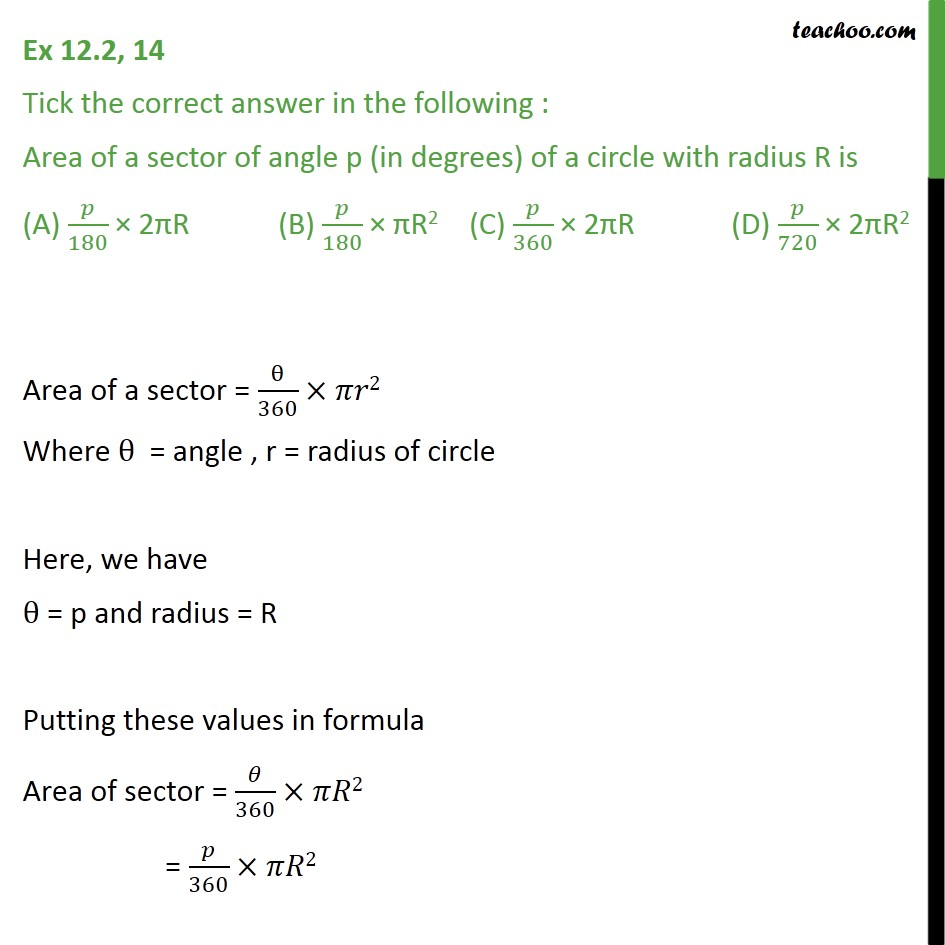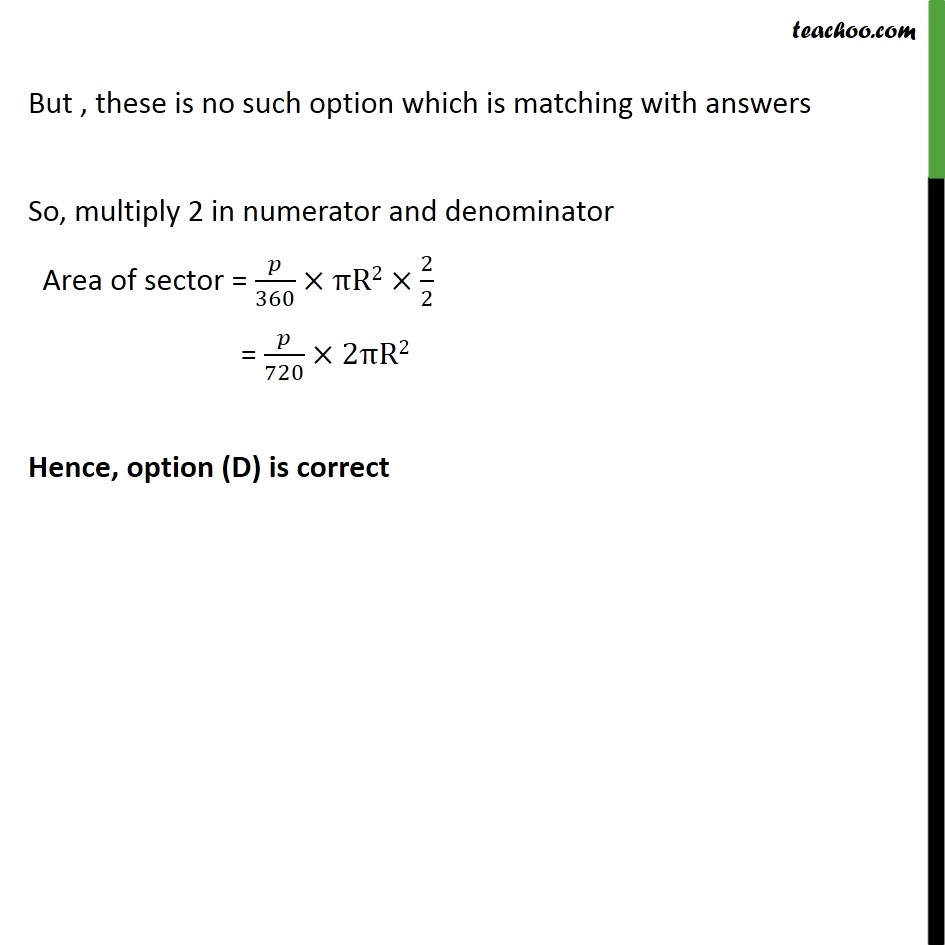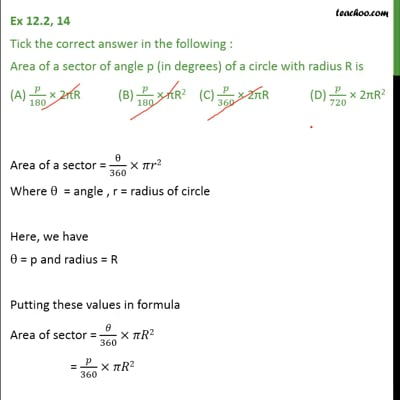Ex 12.2

Chapter 12 Class 10 Areas related to Circles
Serial order wiseThis video is only available for Teachoo black users

Solve all your doubts with Teachoo Black (new monthly pack available now!)

### Transcript

Ex 12.2, 14 Tick the correct answer in the following : Area of a sector of angle p (in degrees) of a circle with radius R is (A) /180 2 R (B) /180 R2 (C) /360 2 R (D) /720 2 R2 Area of a sector = /360 2 Where = angle , r = radius of circle Here, we have = p and radius = R Putting these values in formula Area of sector = /360 2 = /360 2 But , these is no such option which is matching with answers So, multiply 2 in numerator and denominator Area of sector = /360 R2 2/2 = /720 2 R2 Hence, option (D) is correct